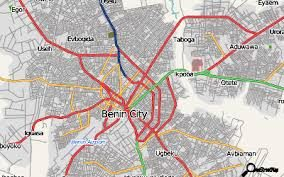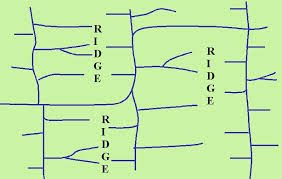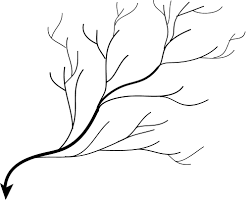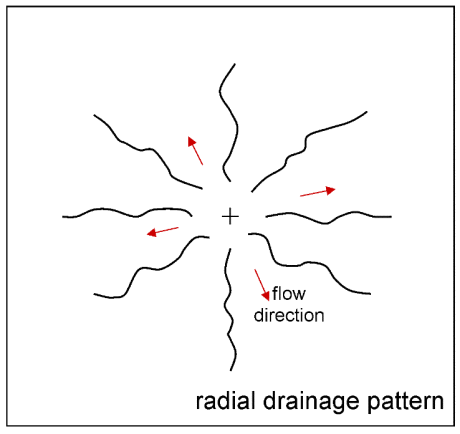# MPA: ANALYSIS OF LINEAR FEATURES

##### This unit is based on the elements of linear features on a map

Measuring the length or distance of a linear feature

Regular and Irregular Line Shapes

It is easier to measure a regular shape than an irregular shape. If a linear feature has a regular shape, that is it is a straight line, a ruler can be used to directly measure its length on the map. On the other hand, if a linear feature has an irregular shape, that is, it is curvy or zigzag in nature, either the edge of a piece of paper or a white thread (the type used by tailors), is used to measure the length of the feature, carefully following the curves. Mark the beginning and the end of the feature on the material you are using to do the measurement. After tracing or measuring the length of the feature on the map using the thread or paper, stretch out the material used over a ruler and read off and record the total length (usually in centimetres, or inches, as the case may be). Next, use the scale of the map to calculate the equivalent distance on ground.

Thus, the logical steps involved in calculating the length or distance of a linear feature are:

• Measure the length of the feature on paper (map). This can be achieved in a couple of ways. If the line is a straight line (as the crow flies) you can use a ruler to measure it, or alternatively you can use a string to measure it and stretch the string on a ruler to read off the length in centimetres. On the other hand, if the line is winding, bend the string to follow the exact shape until you reach the second or end point. Then place the string against a ruler or the scale bar on the map to measure the distance on your string.

• Now that you have a distance in centimeters, using the map scale, calculate the ground equivalent of the distance on paper using the following formula:

MD x MS

Lg    =

Where:

100,000

Lg = Length of a linear feature on ground. (This is usually in kilometres, or miles).

MD = Map distance (length of the feature as measured on the map. This distance is usually measured in centimetres, or inches)

MS =  Map Scale (the denominator of the map scale)

100,000 = a constant used to convert from centimetres to kilometres (NB: 100,000 cm is equal to 1km).

Problem:

If a portion of a road measures 6.3cm on a map at a scale of 1:50000, what is the length of the road portion on ground?

Solution:

MD = 6.3cm

MS = 50000

Therefore,

MD x MS

Lg    =

100,000

3 x 50000

100000

15km (NB: Always indicate the correct unit of measurement of your answer).

Roads connect different settlements in any given geographical area. For planning and development purposes, it is possible and often necessary to determine the level of connectivity in an area. The level connectivity in a place also indicates the degree of accessibility or ease of movement from one location to another in that place. Poor road connectivity in, say a city, means people will find it difficult moving around in that city. The desirable ideal situation, therefore, is to have full or one hundred percent connectivity. However, in reality full connectivity is hardly attained. By calculating the present level of road connectivity, planners and decision makers are able to know the number of additional road networks that might be needed to achieve full connectivity or at least significantly improve on the situation on ground.

Nodes represent settlements while links (also known as arcs) represent road segments connecting the settlements.Drainage Analysis

Drainage here refers to streams and rivers. The drainage resources existing in a place are usually shown on topographic, physical geography, or hydrological maps. Drainage analysis is important for a number of purposes including water resources management, irrigation and other agricultural activities, hydropower supply, and so on.

We normally deal with two kinds of drainage analysis namely qualitative analysis and quantitative analysis. Qualitative analysis basically has to do with description of the physical distribution and patterns of streams/rivers in a given place. On the other hand, quantitative drainage analysis is concerned with issues such as number of streams, bifurcation ration, length of stream, area of stream (or river) basin, stream density, stream frequency, and stream intensity.

Types of Drainage Pattern

There are different types of drainage pattern which can be shown on a map. Some of the major drainage patterns are identified and illustrated below.

Trellis drainage pattern: In a trellis pattern  the distributaries of a stream run almost parallel to each other; hence they join the main stream at almost right angle.Dendritic drainage pattern:  This drainage pattern is similar to the branches of trees; it is characterised by irregular branching of the tributaries of a stream in different directions.Centripetal drainage pattern: This pattern is characterised by many streams or rivers converging or flowing into a lake from different directionsRadial drainage pattern: In a radial drainage pattern several streams or rivers flow from a central highland (hill or mountain) to different directions away from each otherStream Ordering

Having identified and delineated a particular drainage system for analysis, the first thing to do is to carry out a systematic ordering or ranking of the various stream segments making up that drainage system. There are several methods of ranking stream segments. However, for the purpose of this course we will adopt a modified version of the method devised by Strahler. In this method stream segments are ranked as 1st order, 2nd order, 3rd order, and so on. A  first order stream is any stream segment that does not have any other stream flowing into it. When two first order streams meet they form a 2nd order stream. Similarly, when two 2nd order streams meet they form a 3rd order stream, and so on. It should also be noted that as indicated , when a lower order stream joins a higher order stream the resulting stream segment will still be that higher order stream. For instance, if a 1st order stream flows into a 2nd order stream, the stream segment produced will still be ranked 2nd order. In the same vein, if a 2nd order stream joins a 3rd order to produce a new stream segment, this new segment will be ranked 3rd order. It is only when two (or more) stream segments of the same order meet at a common confluence to form another segment that a higher order stream is produced.

Stream ordering helps us to identify and know the number of segments in each order or category, and hence, the total number of segments in the drainage system under consideration. It is, therefore, customary to create a stream order table after ordering the streams in a drainage basin. Table 2 has been generated based on the stream ordering in Figure 3.6; the Table shows the number of segments in each stream order.

Bifurcation ratio (Br) is used to compare the relationship between a stream order and the next higher stream order. In other words, it is used to determine the proportion of a stream order say the nth order (i.e. 1st order) in relation to the (n+1)th order (i.e. 2nd order). The formula for calculating the Bifurcation ratio is:

 Br = Sn Number of stream segments in the nth order = Number of stream segments in the (n+1)th order S(n+1)

Drainage Density (Dd)

This refers to the average or mean length of streams in a drainage basin. To determine this index, the total length of all stream segments in the basin is measured and divided by the area of the basin. This gives us an idea of the average length of stream per unit area; e.g. 3.2km of stream per square kilometre. The stream density is determined by the formula:

Total length of streams

Dd      =                  =

• Area of stream basin

Stream Frequency (Sf)

This is the mean number of stream segments in a drainage basin per unit area of the basin. To obtain this index, simply count the number of stream segments in the basin and divide it by the area of the basin. This will give us an idea of the average number of segments per unit area.

 Total number of stream segments Sf   = = A Area of stream basin

Length Ratio (LR)

This index measures the relationship or ratio between the average length of all the stream segments belonging to a particular stream order and the average length of all the stream segments in the next higher stream order.

LR    =

/ Sn+1Total length of 1st order streams / number of segments in 1st order

Total length of 2nd order streams / number of segments in 2nd order

Drainage intensity (Di)

This is a product of the drainage density and stream frequency. The formula for calculating drainage intensity is:

∑l∑ns

Di    =                         =       DdSf

A2

Where:  Dd = Drainage density

Sf  =  Stream frequency

SEE ALL
•YOU